Email Us: info@lupinepublishers.comCall Us: +1 (914) 407-6109   57 West 57th Street, 3rd floor, New York - NY 10019, USA

# Lupine Publishers Group

## Lupine Publishers

Submit Manuscript

ISSN: 2643-6736# Magnus Force in Inertial Microfluidics-Based Devices Volume 2 - Issue 1

Ali Farajpour1, Mergen H Ghayesh1* and Hamed Farokhi2

• 1School of Mechanical Engineering, University of Adelaide, South Australia, 5005, Australia
• 2Department of Mechanical and Construction Engineering, Northumbria University, Newcastle upon Tyne, NE1 8ST, UK

Received:April 09, 2019;   Published:April 18, 2019

Corresponding author:Mergen H Ghayesh, School of Mechanical Engineering, Australia

## Abstract

In this paper, the influences of Magnus force on the behaviour of small particles inside a microfluidics- based device are theoretically investigated. The Magnus force is a lift force, which is caused by the rotation of particles within fluid flow. Explicit relations are presented for calculating the Magnus force exerted on small particles inside fluid flow with small Reynolds numbers. Moreover, the effects of the radius of a particle on the Magnus force are discussed. The obtained results and formulation would be helpful in the design of inertial microfluidics-based devices.

Keywords:Inertial Microfluidics; Particle Separation; Magnus Force; Microsystems

## Introduction

Microtechnology and nanotechnology [1-8]. have many promising applications in various areas of science and medicine. Particularly, in medicine, novel diagnosis and treatment techniques have been introduced using microscale and nanoscale devices . These techniques are ultra-fast, portable, less costly and easy to use compared to traditional techniques . In addition, in engineering, microscale structures [11-23]. and nanoscale structures [24-31]. have been utilized to fabricate microscale and nanoscale devices such as ultra small actuators, sensors and energy harvesters. Among different microscale systems, inertial microfluidics-based devices have attracted much interest from scientific communities due to their potential for the separation of particles and fluids at ultra-small levels. When a particle travels inside the channel of a microfluidics-based device, it is subject to several forces such as drag, diffusion, Saffman and Magnus forces. In this paper, Magnus force, as one of important forces in a group of microfluidics-based devices, is investigated. A mathematical explicit relation is given for the Magnus force. Furthermore, the effect of particle radius on the Magnus force is studied in detail.

## Magnus Force

In this section, the Magnus force is formulated, and the influence of particle radius on this force is studied. Let us consider a spherical particle within the fluid of constant Velocityand density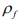(Figure 1). The fluid is assumed to be incompressible and viscous. Furthermore, at the beginning, the particle is symmetrically surrounded by the fluid. The angular velocity and radius of the particle are denoted byand R, respectively. It is assumed that there is no relative motion between the particle and fluid on the interface. When the particle starts to spin as shown in Figure 1, the fluid velocity below the particle is lower than the one above the particle. As a result, the pressure is higher below the particle, and this leads to a lift force. This lift force, which is technically called Magnus force, is calculated by the following relation for small Reynolds numbers .

Figure 1: Magnus Force Exerted on An Arbitrary Particle In A Microfluidics-Based Device For The Separation Of Circulating Tumor Cell.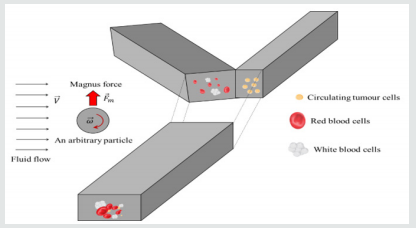where o(Re) is a function of Reynolds number (i.e.). The dynamic viscosity of the

fluid is denoted by. For particles with a very small radius, which is the case in microfluidic devices,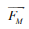is independent of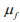. In microfluidic devices, in addition to rotation, particles usually travel along

the channel. Let us consider a small particle, which spins with angular velocityand travelswith velocity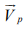inside the fluid. In this case, the Magnus force is given by

in whichand are, respectively, the fluid velocity and relative velocity. Figure 2 shows the variation of the Magnus force with particle radius. The results are calculated for the blood flow with density 1060 kg/m3 and velocity 0.33 mm/s. It is observed that the Magnus force rapidly increases with increasing the radius of the particle. The formula and results of this paper would be useful in the analysis of fluid-conveying ultra small systems such as ultra small tubes conveying fluid [33-39] as well as microfluidicsbased devices [40-42].

## Conclusion

The effect of Magnus force on the motion of particles with relatively small sizes inside an inertial microfluidic device has been examined. Explicit relations were given for determining the Magnus force acting on particles within fluid flow of small Reynolds numbers. It was observed that the Magnus force acts as a lift force and is caused by the rotation of particles. In addition, it was concluded that the radius of the particle has a significant role to play in the Magnus force. The Magnus force substantially increases with increasing particle radius.

Close## Online Submission System

### Submit ManuscriptClick here for Manuscript Sample Templates### Drag and drop files here

or

( For multiple files submission, zip them in a single file to submit. For file zipping software Download )